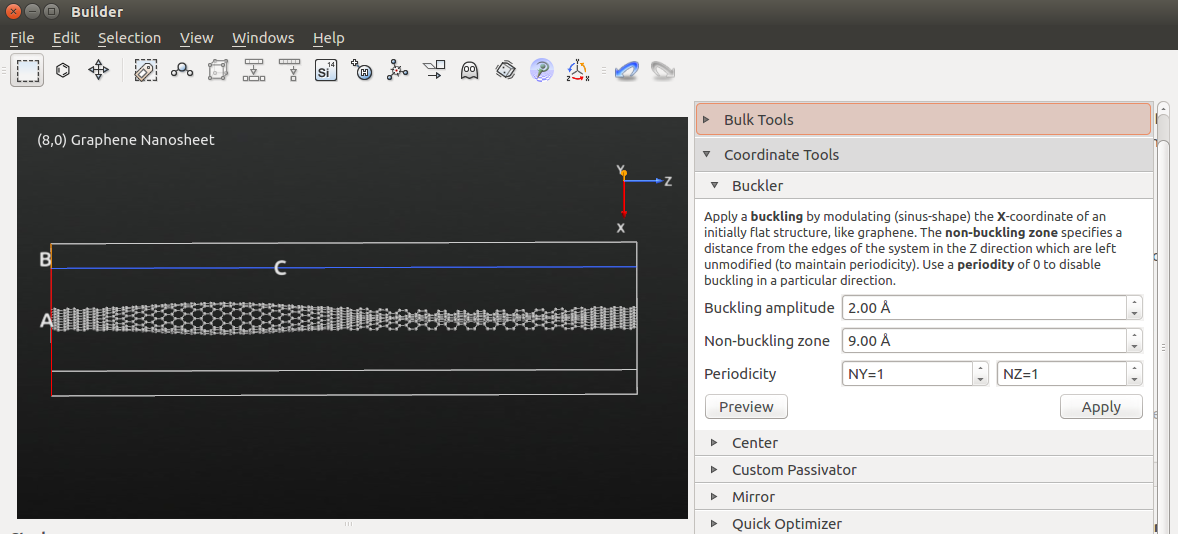# Exploring Graphene¶

In this tutorial you will build a range of graphene structures and study some of their properties. With QuantumATK you can easily twist and stretch the structures using plug-in modules. You can then use QuantumATK to calculate their electronic and vibrational properties. You will also learn to automate calculations on large structures using Python scripting.Hint

In many QuantumATK projects (depending on the study of course) you will need the appropriate plugins/AddOns, which can be installed via the AddOn Manager.

In this particular tutorial, you will need the TubeWrapper.zip and CNTBuilder plugins. Please check that these are available, and install them if they are not. You can install addons following the instructions in the How to create AddOns for QuantumATK page.

## Build a graphene sheet¶

Open the QuantumATK Builder. It is then easy to create a graphene sheet using a plugin; simply click Add ‣ From Plugin ‣ Nanosheet. Leave the option for Chemical Properties at defaults. In the Geometry options, choose a chiral vector of (n,m)=(8,0), and click Build.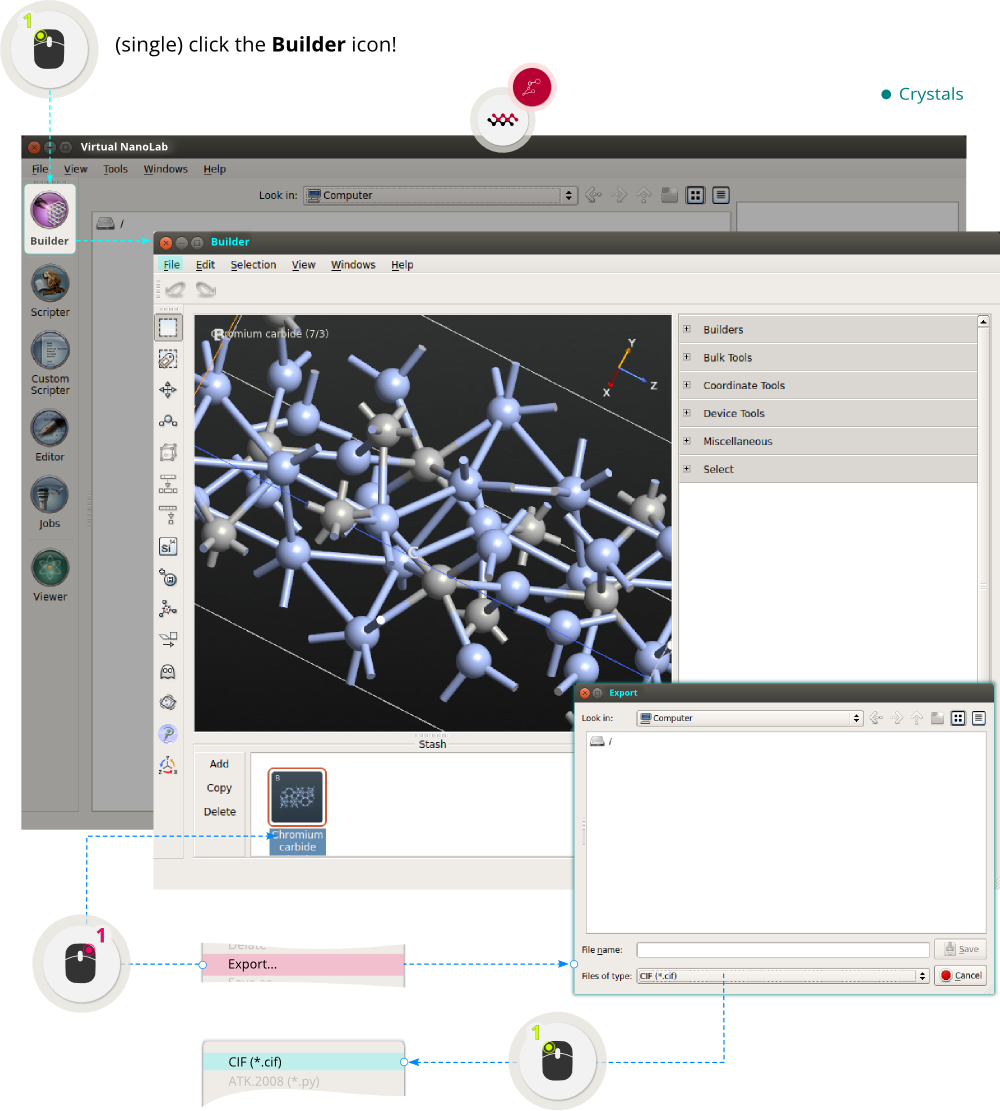You now have a basic “building block” for the structure. Use the Bulk Tools ‣ Repeat plugin to repeat it 10 times along the C-direction:## Build a CNT¶

You can now use the TubeWrapper plugin to wrap the nanosheet into a carbon nanotube (CNT). It is possible to turn the nanosheet into an open or a closed cylinder: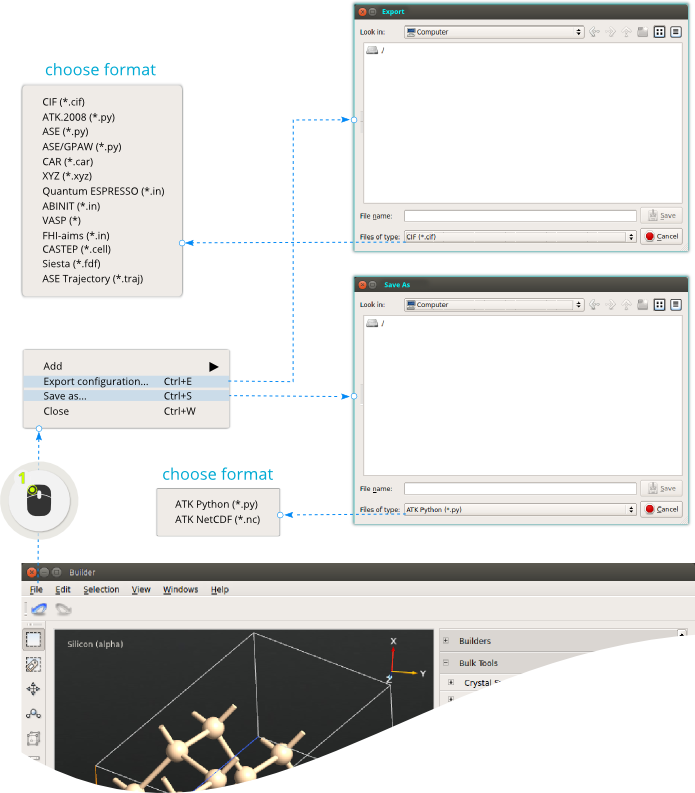## Transmission spectrum of a GNR¶

Let us calculate the electronic transmission spectrun of a graphene nanoribbon (GNR). First, build the ribbon using the Add ‣ From Plugin ‣ Nanoribbon plugin. Choose chiral indices (n,m)=(1,1) and repeat the structure 3 times the C-direction: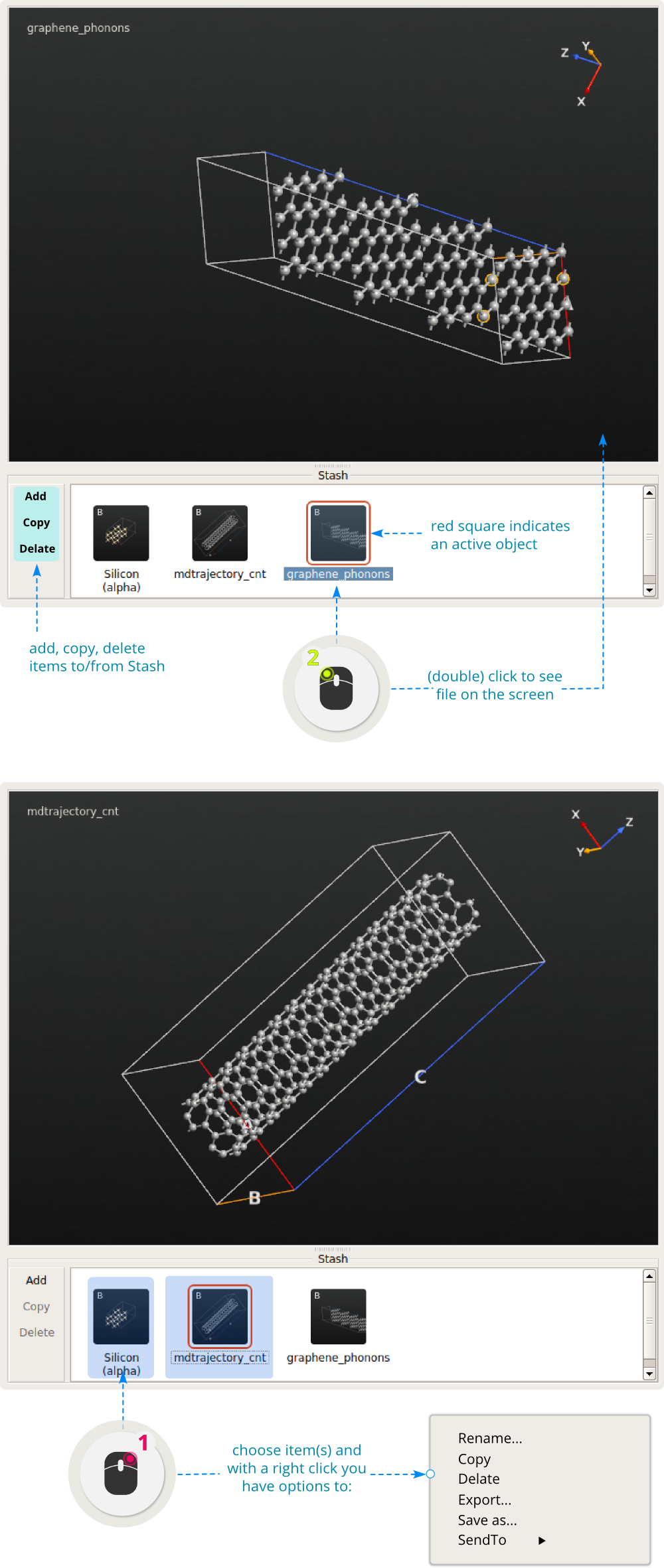Note

In order to correctly calculate the transmission spectrum for a bulk system, the bulk must qualify as a valid device electrode. This requires that the C-axis is perpendicular to the A,B plane, and that it is sufficiently long that the atoms in the unit cell only have Hamiltonian matrix elements with atoms in the nearest neighbour cells along C. This condition is usually fullfilled if the C-vector is longer than 7 Å.

Next, transfer the structure to the Script Generator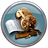using the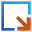botton, and double-click theNew Calculator icon to add a calculator block to the script.

• First, change the default output file to ribbon11_bulk.hdf5.
• Then open the added calculator block to start editing the settings.
• Select the Extended Huckel calculator, and make sure the number of k-points is 1 for the A and B directions, and high for the C-direction, e.g. 100 k-points along C.
• In the Huckel basis set options, select Cerda.Carbon [graphite] for carbon and Cerda.Hydrogen [C2H4] for hydrogen.
• Finally, add aTransmissionSpectrum block to the script, and save the Python script as ribbon11_bulk.py.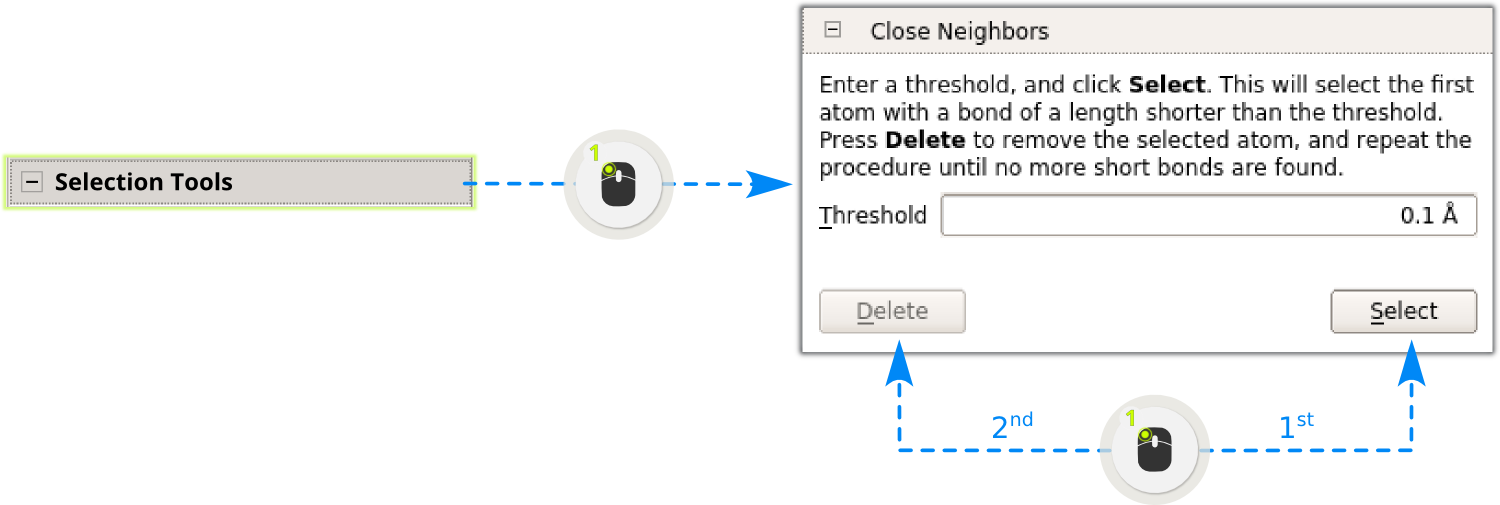Send the script to the Job Manager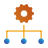in order to execute it. The job starts when you click the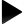icon.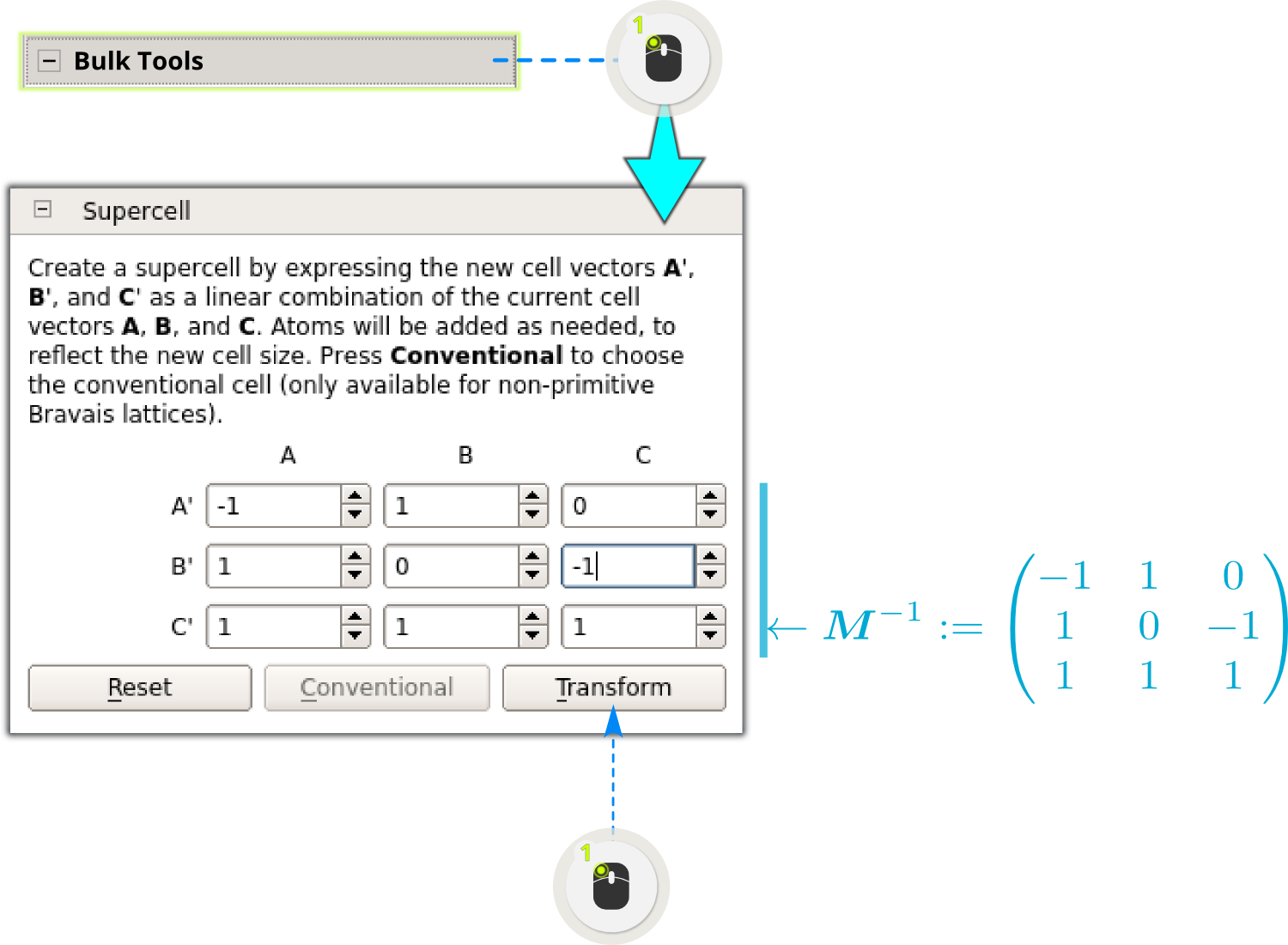When the calculation is finished (it will run extremely fast), locate the output file ribbon11_twist0_nscf.hdf5 in the QuantumATK file browser window. Select the file and notice that the contents of the file are displayed in the QuantumATK panel: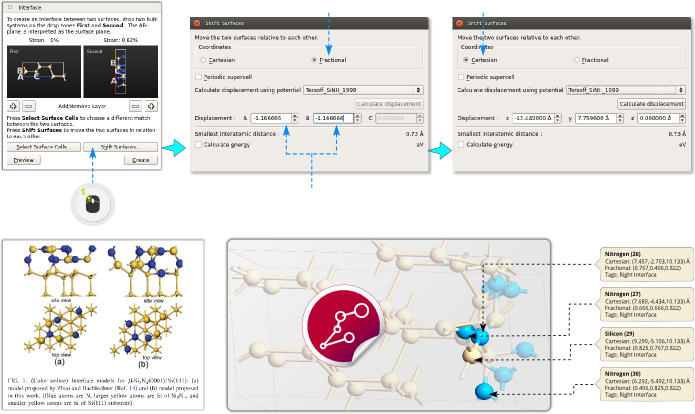You can now select the TransmissionSpectrum object, which has this icon: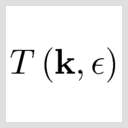and use the 2D Plot or Transmission Analyzer plugins to plot the electronic transmission spectrum.

## Twisted nanoribbon¶

In this section you will learn how to twist a graphene nanoribbon using the QuantumATK Twister plugin, and then compute the transmission spectrum.

Open theBuilder, and select the nanoribbon created in the previous section. Then use the Bulk Tools ‣ Repeat tool to repeat the configuration 7 times in the C direction: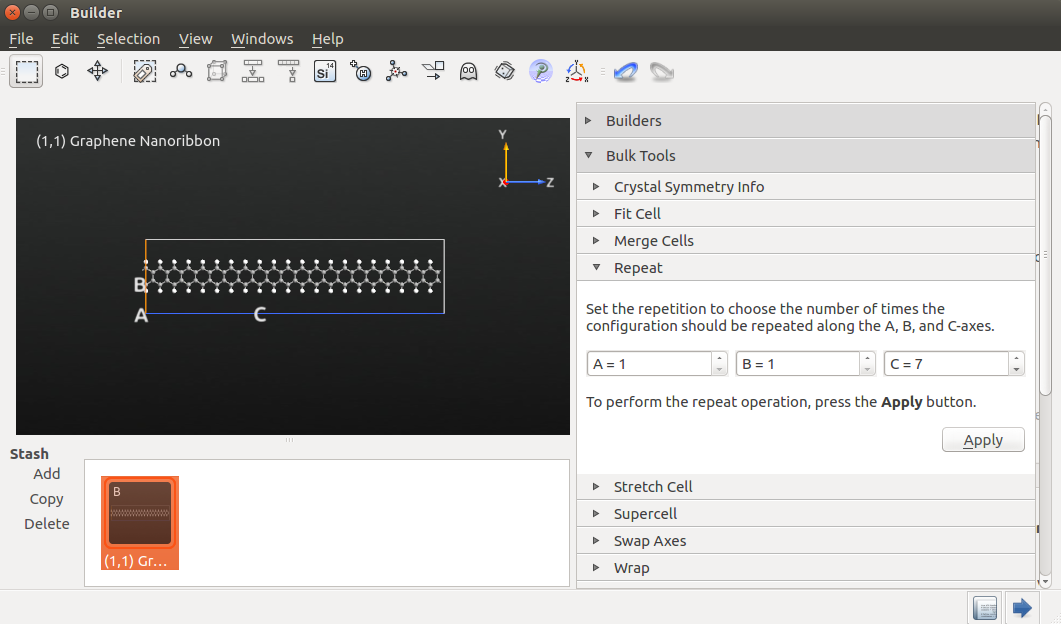Then open the Coordinate Tools ‣ Twister plugin, and set a “Twist angle” of 72 degrees and a “Non-twisting zone” of 9 Å. Click Apply to perform the twisting operation.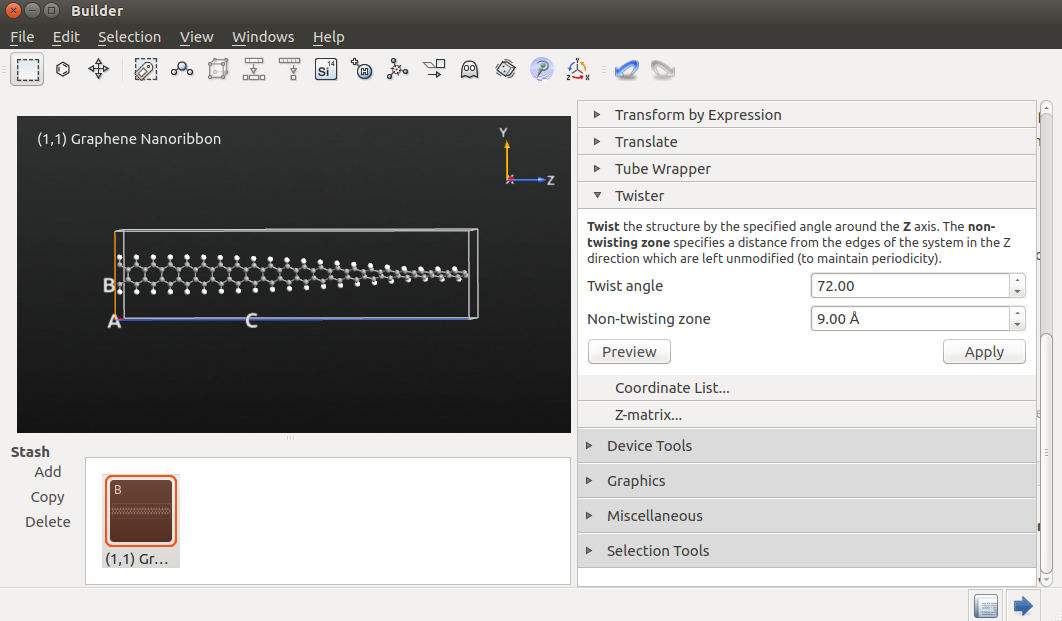Certain parts of the system to the left and right of the structure are not twisted; the length of the non-twisted part is specified by the value of “Non-twisting zone”. The rest of the structure is twisted by the sepcified angle.

### Transmission spectrum¶

You will now calculate the transmission spectrum of the twisted graphene nanoribbon. First, you need to convert the twisteds ribbon into a device – use the Device Tools ‣ Device from Bulk tool for this.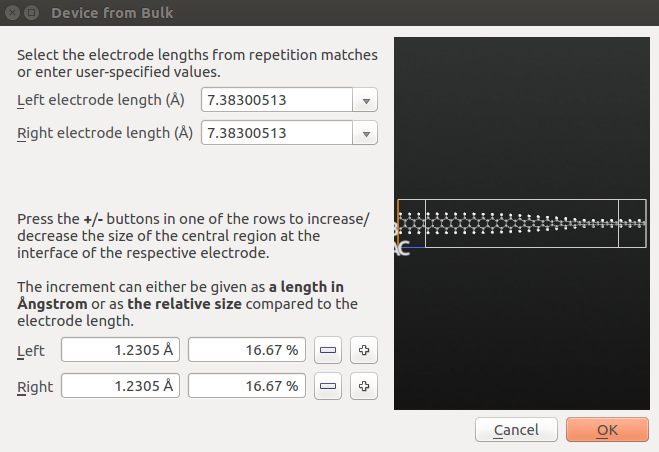Then rename the device configuration as GNR_twist and send it to theScript Generator to create the required ATK Python script.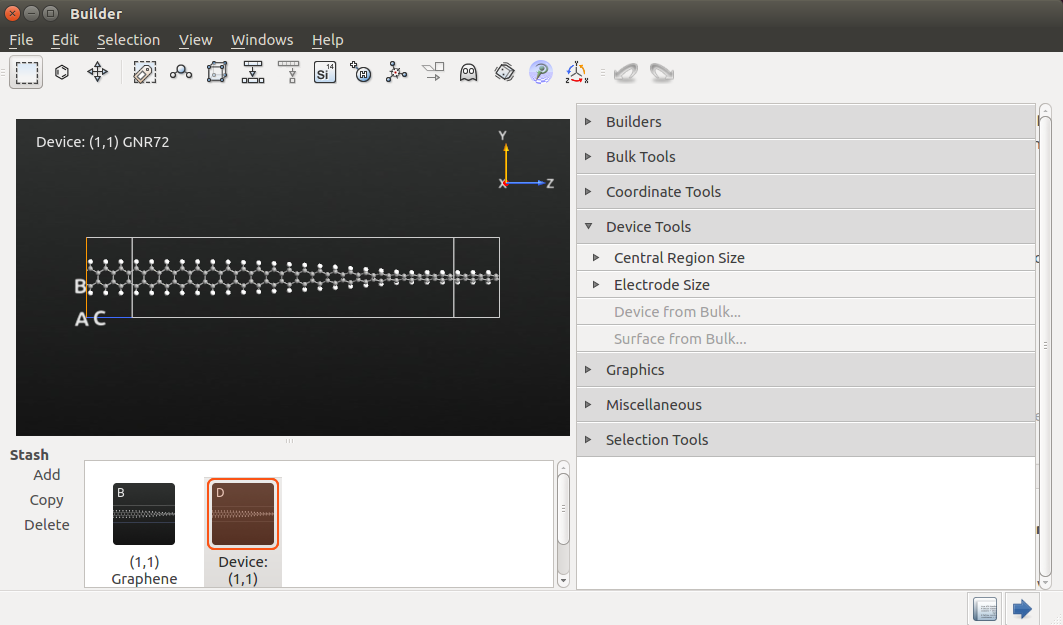In the Scripter, add the following script blocks:

Open the New Calculator block and use the following settings:

• “ATK-SE: Extended Hückel (Device)” calculator;
• 1x1x100 k-point grid;
• “Cerda.Carbon [graphite]” basis set for C and “Cerda.Hydrogen[C2H4]” basis set for H.

Select the following settings in TransmissionSpectrum block:

• Krylov self-energy calculator;
• 1x1 k-point grid.

Then save the script as GNR_twist.py and execute it using theJob Manager. This calculation will take just a few minutes.

The HDF5 data file GNR_twist.hdf5 should now have appeared on the QuantumATK LabFloor. Select the TransmissionSpectrum item and use the Transmission Analyzer plugin to visualize the computed transmission spectrum.Note that the transmission peak has shifted down in energy as compared to the non-twisted ribbon. The twist represents a source of electron scattering, since it breaks the translational symmetry of the non-twisted ribbon electrodes along the transport direction.

## Möbius nanoribbon¶

Next, we are going to create a Möbius nanoribbon. Download the script Moebius.py, which is also reproduced below. The script loads a basic nanoribbon from the file nanoribbon.hdf5, then performs the required operations of repeating, twisting, and wrapping the ribbon, and finally saves the Möbius ribbon in moebius.hdf5.

  1 2 3 4 5 6 7 8 9 10 11 12 13 14 15 16 17 18 19 20 21 22 23 24 25 26 27 28 29 30 31 32 33 34 35 36 37 38 39 40 41 42 43 44 45 46 47 48 49 50 51 52 53 54 55 56 57 58 59 60 61 62 63 64 65 66 67 68 69 70 71 72 73 74 75 76 77 78 79 80 81 82 83 84 85 86 87 88 89 90 91 92 93 from NL.Math.Utilities import rotationMatrix import math from QuantumATK import * def twister_displacement(x, rotation_angle_per_z, rotation_axis, rotation_axis_center, z_start, z_end): """ Function for twisting a 1-d structure @param x : Coordinates of 1-d structure @param rotation_angle_per_z : size of twist in angle/length @param rotation_axis : axis to apply twist along @param rotation_axis_center : center of the rotation axis @param z_start : z value for starting the twist @param z_end : z value for ending the twist """ # do not twist for z > z_end z = x z = min(z,z_end) # do not twist for z < z_start z = z - z_start z = max (z,0.0) # find twist angle theta = z*rotation_angle_per_z # calculate the rotation matrix rotation_matrix = rotationMatrix(theta, *rotation_axis) # apply rotation return rotation_axis_center+numpy.dot(rotation_matrix, x-rotation_axis_center) def wrapping_displacement(x, width, wrapping_angle): """ Function for converting a nanosheet coordinate into a partly wrapped nanotube @param x : Coordinates of nanosheet atom @param width : Width of the nano-sheet @param wrapping_angle : maximum wrapping angle of the nanotube in radians """ # calculate the average radius of the incomplete wrapped tube radius = width/wrapping_angle # find the angle of the current atom angle = (x-width/2.)/radius # calculate the radius of the current atom atom_radius = radius+x # return atom position of the wrapped atom return numpy.array([x, atom_radius*math.cos(angle),atom_radius*math.sin(angle)]) def Moebius(ribbon, n, m, repetition): """ Function for generating a moebius molecule @param n : Chiral vector index @param m : Chiral vector index @param repetition : Repetition along z """ # build n,m ribbon #ribbon = NanoRibbon(n,m) ribbon = ribbon.repeat(1,1,repetition) # get properties of the ribbon lattice = ribbon.bravaisLattice() elements = ribbon.elements() cartesian_coordinates=ribbon.cartesianCoordinates().inUnitsOf(Angstrom) # calculate the length of the 1-d structure z_length = numpy.linalg.norm(lattice.primitiveVectors().inUnitsOf(Angstrom)) # calculate twist parameters rotation_angle_per_z = math.pi /z_length rotation_axis = numpy.array([0,0,1]) rotation_axis_center = numpy.sum(cartesian_coordinates,axis=0)/len(cartesian_coordinates) # define a function of one variable, f(c), for displacing the atoms f = lambda c : twister_displacement(c, rotation_angle_per_z, rotation_axis, rotation_axis_center, 0.,z_length) # apply the function to find new displaced coordinates cartesian_coordinates = numpy.apply_along_axis(f, 1, cartesian_coordinates) cartesian_center = numpy.sum(cartesian_coordinates,axis=0)/len(cartesian_coordinates) cartesian_coordinates = cartesian_coordinates - cartesian_center # define a function of one variable, f(c), for displacing the atoms f = lambda c : wrapping_displacement(c, z_length,2.0*math.pi) # apply the function to find new displaced coordinates cartesian_coordinates = numpy.apply_along_axis(f, 1, cartesian_coordinates) return MoleculeConfiguration( elements=elements, cartesian_coordinates=cartesian_coordinates * Angstrom ) ribbon = nlread('ribbon.nc', BulkConfiguration)[-1] moebius = Moebius(ribbon,1,1,100) nlsave('moebius.nc', moebius) 

You first need to build a basic nanoribbon. Use the Add ‣ From Plugin ‣ Nanoribbon plugin and select, for example, the chiral vector (n,m)=(6,6). Save the created ribbon in the Project Folder as ribbon.hdf5.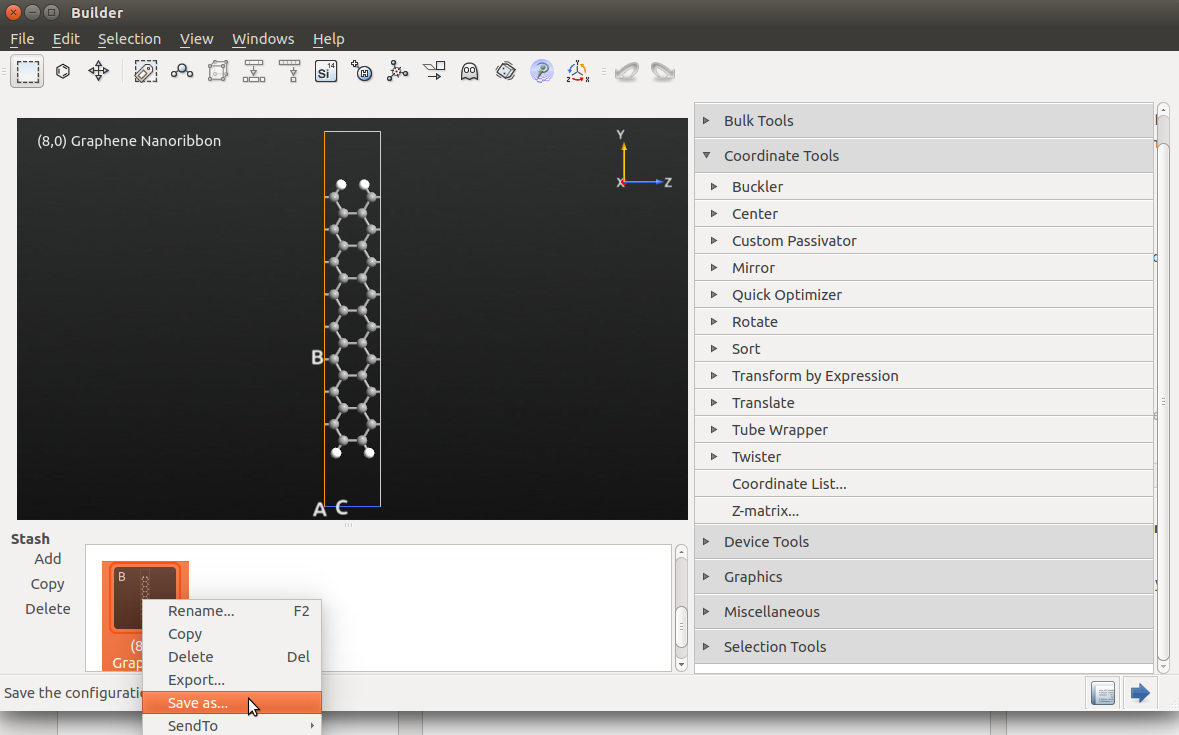Then make sure Moebius.py is also located in the Prpject Folder, and execute it using theJob Manager or from the command line. The output file moebious.hdf5 should appear in the Project Files list and the Möbius configuration should appear on the LabFloor. Use the Viewer to visualize the structure.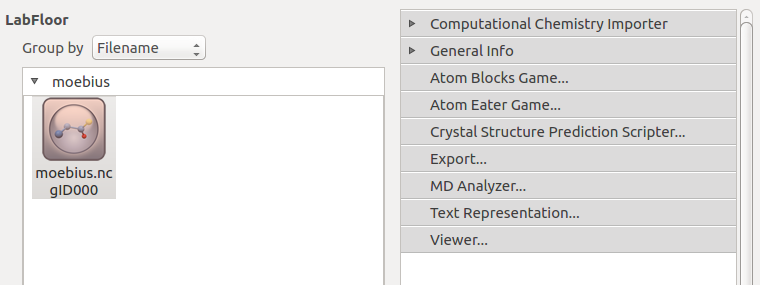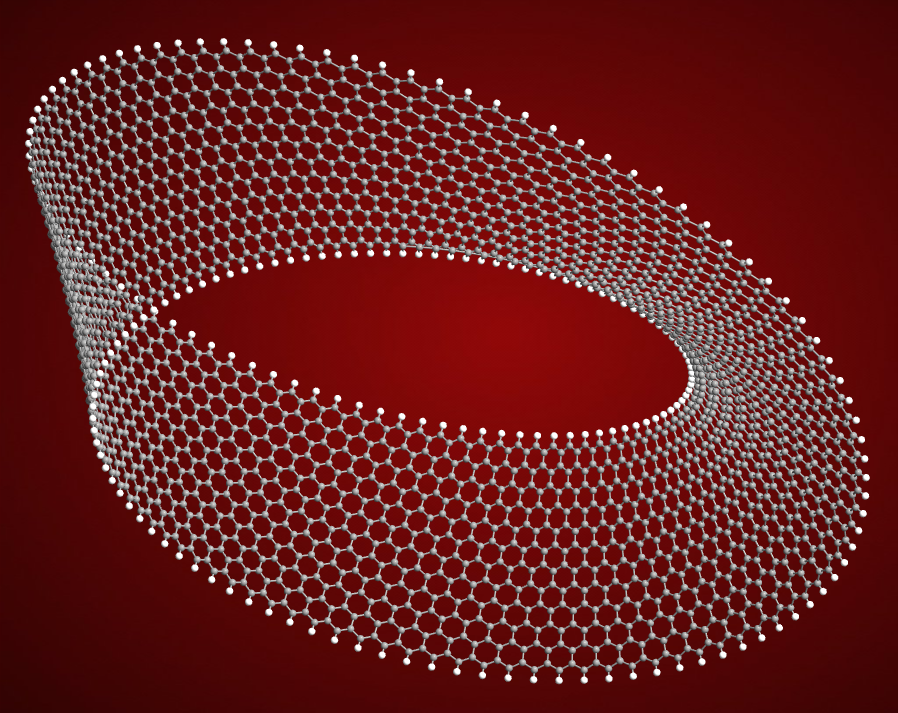## Buckling a graphene sheet¶

In this final section you will learn how to buckle a graphene sheet using the QuantumATK Buckler plugin.

First, create a nanosheet as shown above in section Build a graphene sheet. This time, repeat the nanosheet 30 times in the C direction.

Then open the Coordinate Tools ‣ Buckler plugin, and set the “Buckling amplitude” to 2 Å and the “Non-buckling zone” to 9 Å. Select “NY = 1” and “NZ = 1” to enable buckling along the Y and Z axes.

Click Apply to perform the buckling operation.